Home > GC > Chapter 8 > Lesson 8.1.5 > Problem8-50

8-50.
1. Without using your calculator, find the exact values of x and y in each diagram below. Homework Help ✎

1.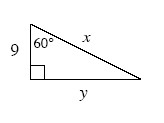2.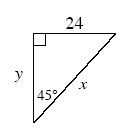3.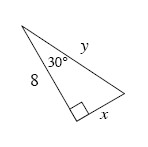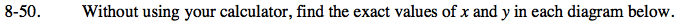All three triangles are special right triangles. See the Math Notes box in Lesson 5.3.1 for shortcuts to find the lengths of sides of special right triangles.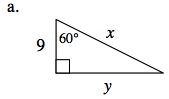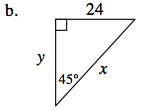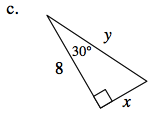$x=18,\ y=9\sqrt{3}$

$x=24\sqrt{2},\ y=24$

Refer to parts (a) and (b).